Get inspired by the success stories of our students in IIT JAM MS, ISI  MStat, CMI MSc DS.  Learn More

# ISI MStat PSB 2004 Problem 1 | Games and ProbabilityThis is a very beautiful sample problem from ISI MStat PSB 2004 Problem 1. Games are best ways to understand the the role of chances in life, solving these kind of problems always indulges me to think and think more on the uncertainties associated with the system. Think it over !!

## Problem- ISI MStat PSB 2004 Problem 1

Suppose two teams play a series of games, each producing a winner and a loser, until one team has won two or more games than the other. Let G be the number of games played. Assume echs team has a chance of 0.5 to win each game, independent of the results of the previous games.

(a) Find the probability distribution of G.

(b) Find the expected value of G.

### Prerequisites

Naive Probability

Counting priciples

Geometric distribution.

Conditional expectation .

## Solution :

While solving this kind of problem, First what we should do is to observe those things which remains invariant.

Here, observe that the game will always terminate, with consecutive wins of one team. Imagine there are two teams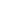and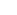. If the first two matches are won by any of the two teams we are done, but saywon the first match, butwon the 2nd match, so its a draw and the 2 matches played are basically lost, and we should renew the counting a fresh.

So, can I claim that G (as defined in the question), will always be even !! verify this claim yourself !

so, considering the event G=g , g is even. So, if the game terminates at the gth game, then it is evident from the logic we established above, then both the (g-1)th and gth game was won by the winning team. So, among the first (g-2) games, both the team won equal number of games, and ended in a draw. So, after the (g-2)th game, the teams can be at a draw in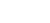ways, and the last two matches can be won by any of the two teams in 2 ways. And the g matches can result in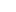different arrangements of wins and loss (from a perspective of any of the teams ).

(a) So, P(G=g)=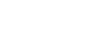; g=2,4,6,.......

Hence the distribution of G. Hold on ! is is looking like geometric somehow ?? Find it out!!

(b) While finding expectation of G, we can use the conventional definition of expectation and, and since I said that distribution of G is (somewhat) geometric, basically to be precise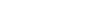, so, clearly expectation will be 4. But I will take this chance to show another beautiful and elegant method to find out expectation, using conditional expectation. So, we will first condition on the win over first match and develop a recursion, which I'm obsessed with. Though one may not find this method useful in this problem since the distribution of G is known to us, but life is not about this problem, isn't it ! What if the distribution is not known but the pattern is visible, only if you are ready to see it. Lets proceed,

Suppose, without loss of generality, let us assume,won the first game, so 1 game is gone with probability 0.5 and with same probability it is expected that E(G|is leading by 1 game ) number of games is to be played, similarly( OR) ifwins the first game, then with probability 0.5 , 1 game is gone and an expected E(G|is leading by 1 game) number of games is to be played.

So, if we right the above words mathematically, it looks like,

E(G)= P(wins the 1rst game )( 1+E(G|is leading by 1 game))+P(wins the 1rst game)(1+E(G|is leading by 1 game)),......................(*)

So, now we need to find out E(G|is leading by 1 game), the other is same due to symmetry!

so, expected number of games to be played when we know thatis leading the by 1 game, is the next game can be a win for, with probability 0.5, OR,can lose the game with probability 0.5, and reach the stage of draw, and from here, all the games played is wasted and we start counting afresh (Loss of memory, remember !! ) , so 1 game is lost and still a expected E(G) numbers of games to follow before it terminates. So, mathematically,

E(G|is leading by 1 game)= P(wins again)x1 + P(looses and draws)(1+E(G))=0.5+0.5+(0.5)E(G)=1+(0.5)E(G).

plugging in this, in (*), one will obtain a recursion of E(G), and calculate is as 4. So, on an average, the game terminates after 4 matches.

## Food For Thought

Can you generalize the above problem, when I say, that the chance of winning each match, for one of the two team is some p, ( 0<p<1) ? Try it !

Wait!! Before leaving, lets toss some coins,

You toss a fair coin repeatedly. What is the expected number of tosses you think, you have to perform to get the pattern of HH (heads and heads) for the first time? What about the expected number of tosses when your pattern of interest is TH (or HT) ?? for which pattern you think you need to spend more time?? Does your intuition corroborates, the mathematical conclusions?? if not what's the reason you think, you are misled by your intuition ??

Think it over and over !! You are dealing with one of the most beautiful perspective of uncertainty !!

## Subscribe to Cheenta at Youtube

This is a very beautiful sample problem from ISI MStat PSB 2004 Problem 1. Games are best ways to understand the the role of chances in life, solving these kind of problems always indulges me to think and think more on the uncertainties associated with the system. Think it over !!

## Problem- ISI MStat PSB 2004 Problem 1

Suppose two teams play a series of games, each producing a winner and a loser, until one team has won two or more games than the other. Let G be the number of games played. Assume echs team has a chance of 0.5 to win each game, independent of the results of the previous games.

(a) Find the probability distribution of G.

(b) Find the expected value of G.

### Prerequisites

Naive Probability

Counting priciples

Geometric distribution.

Conditional expectation .

## Solution :

While solving this kind of problem, First what we should do is to observe those things which remains invariant.

Here, observe that the game will always terminate, with consecutive wins of one team. Imagine there are two teamsand. If the first two matches are won by any of the two teams we are done, but saywon the first match, butwon the 2nd match, so its a draw and the 2 matches played are basically lost, and we should renew the counting a fresh.

So, can I claim that G (as defined in the question), will always be even !! verify this claim yourself !

so, considering the event G=g , g is even. So, if the game terminates at the gth game, then it is evident from the logic we established above, then both the (g-1)th and gth game was won by the winning team. So, among the first (g-2) games, both the team won equal number of games, and ended in a draw. So, after the (g-2)th game, the teams can be at a draw inways, and the last two matches can be won by any of the two teams in 2 ways. And the g matches can result indifferent arrangements of wins and loss (from a perspective of any of the teams ).

(a) So, P(G=g)=; g=2,4,6,.......

Hence the distribution of G. Hold on ! is is looking like geometric somehow ?? Find it out!!

(b) While finding expectation of G, we can use the conventional definition of expectation and, and since I said that distribution of G is (somewhat) geometric, basically to be precise, so, clearly expectation will be 4. But I will take this chance to show another beautiful and elegant method to find out expectation, using conditional expectation. So, we will first condition on the win over first match and develop a recursion, which I'm obsessed with. Though one may not find this method useful in this problem since the distribution of G is known to us, but life is not about this problem, isn't it ! What if the distribution is not known but the pattern is visible, only if you are ready to see it. Lets proceed,

Suppose, without loss of generality, let us assume,won the first game, so 1 game is gone with probability 0.5 and with same probability it is expected that E(G|is leading by 1 game ) number of games is to be played, similarly( OR) ifwins the first game, then with probability 0.5 , 1 game is gone and an expected E(G|is leading by 1 game) number of games is to be played.

So, if we right the above words mathematically, it looks like,

E(G)= P(wins the 1rst game )( 1+E(G|is leading by 1 game))+P(wins the 1rst game)(1+E(G|is leading by 1 game)),......................(*)

So, now we need to find out E(G|is leading by 1 game), the other is same due to symmetry!

so, expected number of games to be played when we know thatis leading the by 1 game, is the next game can be a win for, with probability 0.5, OR,can lose the game with probability 0.5, and reach the stage of draw, and from here, all the games played is wasted and we start counting afresh (Loss of memory, remember !! ) , so 1 game is lost and still a expected E(G) numbers of games to follow before it terminates. So, mathematically,

E(G|is leading by 1 game)= P(wins again)x1 + P(looses and draws)(1+E(G))=0.5+0.5+(0.5)E(G)=1+(0.5)E(G).

plugging in this, in (*), one will obtain a recursion of E(G), and calculate is as 4. So, on an average, the game terminates after 4 matches.

## Food For Thought

Can you generalize the above problem, when I say, that the chance of winning each match, for one of the two team is some p, ( 0<p<1) ? Try it !

Wait!! Before leaving, lets toss some coins,

You toss a fair coin repeatedly. What is the expected number of tosses you think, you have to perform to get the pattern of HH (heads and heads) for the first time? What about the expected number of tosses when your pattern of interest is TH (or HT) ?? for which pattern you think you need to spend more time?? Does your intuition corroborates, the mathematical conclusions?? if not what's the reason you think, you are misled by your intuition ??

Think it over and over !! You are dealing with one of the most beautiful perspective of uncertainty !!

## Subscribe to Cheenta at Youtube

This site uses Akismet to reduce spam. Learn how your comment data is processed.

### 6 comments on “ISI MStat PSB 2004 Problem 1 | Games and Probability”

1.abhishek sinha says:

Part (b) can be easily solved by Optional Stopping time theorem, by considering the Martingale sequence (S_n^2-n). We have E(T)= E(S_T^2)=4.

1.Uttaran Chatterjee says:

Martingale sequence is not included in the syllabus for MStat entrance! And I acknowledged that there is more trivial solution to part (b), which i even wrote, at first, but my point was to generate intuitions and show how these kind of problems can also be handled by conditioning (on wishful thinking) and ending up with a recursion, that was the motive behind providing an elaborate solution.

2.abhishek sinha says:

Martingales provide an elegant (and one line) solution to these problems. We don't even need to find the distribution of the stopping time to compute its expectation.

3.Himanshu Gupta says:

I may sound totally irrelevant.
So, you assumed that G is even in all conditions. But if let's first game is won by the first-team T_1 and second is lost by T_1 means T_2 and then on the third game if T_2 win again then total games are 3. This question didn't mention explicitly that games need to happen in blocks of 2 at a time.
Can you fill me in?

1.Uttaran Chatterjee says:

It clearly says to win the series one team must lead by 2 or more games.
The case you illustrated, is not the situation the game gets terminated, just one of the team is leading by 1 game, but that is not sufficient to conclude ta game, so the game may terminate in the 4th match if the leading team wins, or it may continue to end up in a draw and hence we are again back at square 1. And that proves my claim to be correct!

4.Himanshu Gupta says:

Got it thanks

### Knowledge Partner